# Mandelbrot Set and Friends

(Some rambling discussion and one new picture at mandelbrot-d.html.)

Mandelbrot set: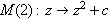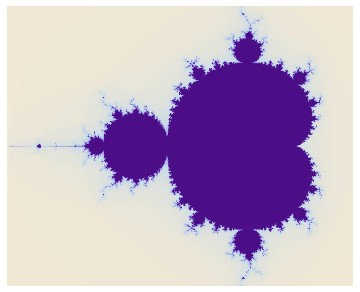Mandelbar set: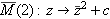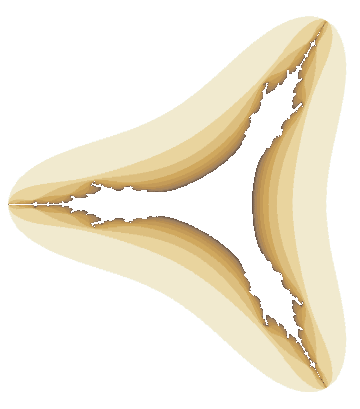Mandelbrot(3) set: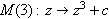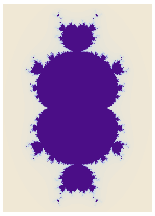Mandelbar(3) set: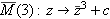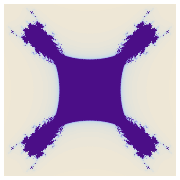Mandelbrot(4) set: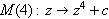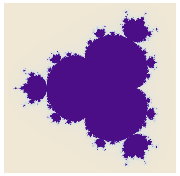Mandelbar(4) set: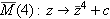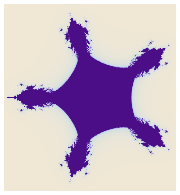Following is the code for the Mandelbrot set (in short, for a 175-by-175 grid of complex numbers c (written as x + i y here) values from -2.01 - 1.1i to 0.7 + 1.1i, we iterate zz2 + c until |z| > 2 or we’ve gone through 50 iterations):

```DensityPlot[
-Length[
FixedPointList[ #^2 + (x + I y) &, x + I y, 50,
SameTest->(Abs[#2] > 2.0 &) ]],
{x, -2.01, 0.7}, {y, -1.1, 1.1},
PlotPoints -> 175, Mesh -> False, Frame -> False,
AspectRatio -> Automatic ];```

For the other pictures, change #^2 to #^3, etc., or to Conjugate[#]^3, etc. In Mathematica 3.0 we can make the function more than five times faster by compiling the iteration function. Here’s the compiled version (which I haven’t actually tried since 1997):

```cMandelbrot =
Compile[{{c, _Complex}},
-Length[
FixedPointList[#^2 + c &, c, 50,
SameTest -> (Abs[#2] > 2.0 &)]]];

ListDensityPlot[
Table[cMandelbrot[a + b I],
{b, -1.1, 1.1, 0.0114}, {a, -2.0, 0.5, 0.0142}],
Mesh -> False, AspectRatio -> Automatic, Frame -> False];```

Designed and rendered using Mathematica 2.2 for the Apple Macintosh and (much, much later) 7.0 for Microsoft Windows.

M-notation and general inspiration lifted long ago from Alexander, Giblin, and Newton, “Symmetry Groups of Fractals”, The Mathematical Intelligencer, vol. 14, no. 2, Spring 1992.